# Orthogonalization method

A method for solving a system of linear algebraic equations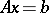with a non-singular matrixbased on the Gram–Schmidt method of orthogonalization of a vector system.

If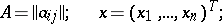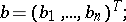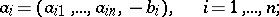then the initial system of equations can be written in the formThis means that the solution of the system is equivalent to the determination of a vectorwhich has 1 as the last component and is orthogonal to all vectors,. For this purpose, an orthogonalization process is used for the vector system, where, which is linearly independent by virtue of the non-singularity of the matrix. This process entails the construction of an orthonormal vector system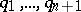, with respect to the scalar product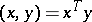, using the recurrence relations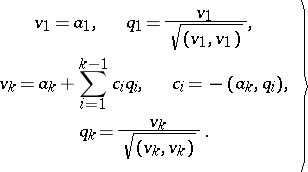(*)

The coefficientsare obtained from the condition of orthogonality of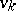to the vectors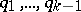. The vectors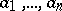are linearly expressed in terms of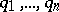, so the vectoris orthogonal to all vectors. The non-singularity ofensures moreover that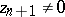. Thus,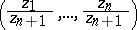is the required solution of the system.

This scheme of the orthogonalization method fits well into the general scheme of direct methods for solving a system: The relations (*) are equivalent to the transformation of the matrix of the system to the matrix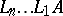, where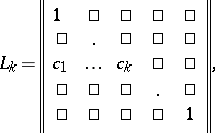and thereby realizes a factorization of the matrix of the system in the form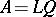, whereis a triangular anda unitary matrix.

The process of factorizing a matrixby means of the orthogonalization method is stable for rounding errors. If in (*), when the operation of taking the scalar product of vectors is carried out, a procedure of accumulation with double precision is used, then for the factorization of the matrix by means of the orthogonalization method one of the best estimates of accuracy in the class of direct methods is obtained. In this case, however, the property of orthogonality of the vectors, i.e. the property thatis unitary, is unstable in relation to rounding errors. So the solution of the system obtained from the recurrence relations (*) can contain a large error. To eliminate this deficiency, various methods of re-orthogonalization are used (see , ).

The speed of operation of the orthogonalization method is inferior to that of many direct methods.